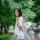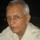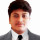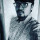CrazyEngineers V4: Early Access

We are developing the next version of CrazyEngineers. If you wish to receive latest updates and early access, click the link below.

1. Home >
2. Apps >
3. Groups >

# If 8+4 = 212, 9+3 = 312, 4+2 = 26, 8+2 = 410 then what is 5+1 = ?

Question asked by Rashmi Gandhi in #Brainy Puzzles on Jun 8, 2019Rashmi Gandhi · Jun 8, 2019
Rank C3 - EXPERT

This was a puzzle from a Kenyan bank. Can you find the correct answer? Do support your answers with explanation of how you came up with it..

WILL post more soon!

Posted in: #Brainy PuzzlesRamani Aswath · Jun 8, 2019
Rank A1 - PRO

a+b=(a/b)(a+b)

8+4=(8/4)(8+4)=212

9+3=(9/3)(9+3)=312  and so on.

So, 5+1=(5/1)(5+1)=56Kaustubh Katdare · Jun 8, 2019
Rank A1 - PRO

Impressive. I've always sucked at solving such problems. ?Ramani Aswath · Jun 8, 2019
Rank A1 - PRO

@Kaustubh Katdare , you’re too modest. I have seen you cracking some tough nuts in a snap of fingers.Rashmi Gandhi · Jun 13, 2019
Rank C3 - EXPERT

Wow! Looks like that's the correct solution. ?Ramya Bhandaru · Feb 3, 2020
Rank D2 - MASTER

8+4=212, 9+3 = 312, 4+2 = 26, 8+2 = 410 then what is 5+1 = ?

the another way of solving this is

8+4=212

this is explained as the first number is divided by second number and placed in the first digit of answer and 2 numbers are added and placed in second and third digits

8/4=2 and 8+4=12 that is 212

9/3=3 and 9+3=12 that is 312

soon...

5+1= then5/1=5 and 5+1=6Venkat Sai Nakkina · Feb 3, 2020
Rank C3 - EXPERT

8+4 = 212, 9+3 = 312, 4+2 = 26, 8+2 = 410 then what is 5+1 = ?

8/4 is 2 and 8+4 is 12 so 8+4=212

9/3 is 3 and 9+3 is 12 so 9+3=312

In similar 5/1 is 5 and 5+1 is 6 so 5+1=56Swarup Pan · Feb 14, 2020
Rank C2 - EXPERT

If 8+4 = 212, 9+3 = 312, 4+2 = 26, 8+2 = 410 then what is 5+1 = ?

The right answer for this question would be.

56Karshil Sheth · Feb 15, 2020
Rank C2 - EXPERT

8,4=12            8/4=2

9,3=12            9/3=3

8,2=10            8/2=4

5,1=6              5/1=5

so Last digit will be 6.

first digit will be 5. so 56

## You must log-in or sign-up to reply to this post.

Click to Log-In or Sign-Up